# 基于多维度空间分配算法实现APP任务定价

## 摘要

本文是针对“拍照赚钱”APP任务定价问题进行分析，并采用多维度空间任务定价模型、会员抉择概率模型、项目总收益模型、入包威胁矩阵模型等对不同情况下的任务进行合理定价。
针对问题一：首先对使用Geoq平台对任务以及会员数据进行定位，在这些数据中发现有部分的异常数据。在对异常数据进行删除处理之后使用任务-会员空间分布模型对其进行合理分配，从而进行数据整合操作，进而使用Python中的Matplotlib工具绘制多功能图来分析任务定价的规律以及部分任务未完成的原因，最终对任务定价数据进行指数拟合。
针对问题二：在该问题中需要制定新的任务定价方案并与原方案进行比较。在完成任务一的前提下，我们首先使用Matlab工具并结合矩阵相关知识对任务-会员进行再次空间分配，之后使用Z-Score对数据进行标准化，进而逐步构建多维度空间任务定价模型、护院抉择概率模型、项目总收益模型来制定新的方案。我们得到结论：任务完成度提高了 12.005% 12.005 % $12.005\%$，项目实际收益提高了 18.3875% 18.3875 % $18.3875\%$，总值为 42964 42964 $42964$
针对问题三：在考虑对任务进行打包的情况下，我们考虑到了会员信誉值和预定任务限额对任务定价的影响，对此构建入包威胁矩阵模型来对各项任务进行打包并将多维度空间任务定价模型进行有话啊，最终基于打包方案的优化模型制定新的方案，求解后得到结论：任务完成率再次提高了 9.341% 9.341 % $9.341\%$，收益率提高了 13.641% 13.641 % $13.641\%$，实际收益总值提高至 45163 45163 $45163$

针对问题四：

## 一、问题重述

### 1.1 问题引言

随着互联网领域的快速发展以及人们对科学技术的不断创新，最近一款称为“拍照赚钱”的服务模式正在逐渐融入到人们的生活中。“拍照赚钱”是移动互联网下的一种新型的自助式服务模式。用户通过下载APP并且注册成为APP的会员之后，就可以在APP平台上领取需要拍照的任务（比如上超市去检查某种商品的上架情况），进而赚取APP对任务所标定的酬金。而这种基于移动互联网的自助式劳务众包平台相对于传统的市场调查方式来说，不仅为企业提供各种商业检查和信息搜集渠道，而且有效地保证了调查数据真实性，缩短了调查的周期，进而可以大大节省人力、物力、财力。因此APP成为该平台运行的核心，而会员接受任务的可能性主要由任务价格决定，故APP中的任务定价是核心要素，而且对于公司的来说起到一个决定性作用。如果任务定价不合理，有的任务就会无人问津，而导致商品检查的失败。相反，若能通过以往数据来对其进行合理性的建模从而确定任务的定价则会取得不凡的结果。

### 1.2 题目数据及信息说明

建模命题组给出的附件一是一个已结束项目的任务数据，其中包含了每个任务的所在地的经纬度、任务的标价以及任务的完成情况（“1”表示完成，“0”表示未完成）；附件二则是会员的信息数据，其中包含了会员所在地的经纬度、预定任务的限额、预定任务的时间和会员所对应的信誉值，而信誉值往往是通过以往的任务完成情况所直接决定的，所以理论上说会员对应的信誉值越大，越优先开始挑选任务，其配额也就越大（任务分配时实际上是根据预订限额所占比例进行配发）；附件三是一个新的检查项目任务数据，其中只有任务所在地的经纬度数据，我们要做的是通过给出的数据来建立一个或若干个模型，进而将此模型应用在这些新项目上从而给平台公司提供一个比较优秀的决策。

### 1.3 提出问题

• 研究附件一中项目的任务定价规律，分析任务未完成的原因。
• 为附件一中的项目设计新的任务定价方案，并和原方案进行比较。
• 实际情况下，多个任务可能因为位置比较集中，导致用户会争相选择，一种考虑是将这些任务联合在一起打包发布。在这种考虑下，如何修改前面的定价模型，对最终的任务完成情况又有什么影响？
• 4.对附件三中的新项目给出你的任务定价方案，并评价该方案的实施效果。

## 二、问题分析

### 2.1 问题的总体分析及思维导图

APP平台中任务定价的设计问题就是通过已完成任务的数据来合理有效的预测未完成任务的执行情况，并使得最终所有任务完成的总价格尽可能大，从而实现APP平台的最大价值率。对此，首先我们需要通过附录一和附录二中的数据来确定影响任务定价的可能相关因素以及分析最终未完成任务的可能原因，以便为后期的建模提供较优的铺垫；然后，在问题二中，我们需要在完成问题一的情况下，对该项目的任务设计一个新的定价方案并建立较优的数学模型，然后可以通过此模型来比较该新的设计方案与原方案的完成情况，进而总结改进之后方案的优点；此外，在问题三中，命题组提到多个集中的任务可能会对用户的选择造成影响，所以我们需要对之前的设计方案进一步通过将这些集中任务进行联合打包发布的方式来对模型进行优化，进而分析最终任务的完成情况；最后在问题四中，我们需要对通过前三问得出的模型来对附录三中的待定任务进行估价并且评价方案的实施效果。

### 2.2 问题的具体分析

#### 2.2.1 问题一

常言有道：具体问题具体分析。对于问题一来讲，我们可以将任务定价的影响因素归于两个方面，一种是已知提供的因素，另一种则是未知潜在的因素。在未知潜在的因素中有任务本身所对应的初始价值以及公司发布任务所在时间点的经营状况等方面因素决定，然而这些因素所决定的条件及信息我们无从得知，因此在这里我们在该论文中不做分析。而在已知提供的因素中，我们主要可以从命题组给出的附录中出发。在给出的附录一和附录二中，我们可以发现其中具有分析价值的数据，对此我们将影响任务的定价初步归因于任务的地理位置、会员所在地的集中程度、任务发布时间以及任务发布地的经济状况四个因素，后期我们也是从这四个因素出发来确定四个因素与任务定价的真正关系。而任务未完成的原因主要从任务定价的合理性、任务的竞争力两个方向进行分析。

#### 2.2.2 问题二

“拍照赚钱”APP的任务定价是一种任务发布者（APP平台）与任务接受者（平台会员）之间所进行的互利模式，PP平台希望在成本一定的情况下使得任务的完成率尽可能的提高，而APP会员则需要在满足某种条件要求下追求收益尽可能大。从实际出发，一份任务理论上可以分配给多个使其相互竞争，而一个会员亦可接受多份任务，这两者在初始阶段是一种“多对多”的关系。而最终的情况是一个任务只能分配给一个会员，而一个会员依然可以接受多份任务，这是一种“多对一”的关系。

#### 2.2.3 问题三

在本问中，提到实际情况下多个任务可能会因为位置比较集中而导致用户会争相选择，为此我们需要采用一种打包方式来对原方案进行优化。在采用任务打包方案之后之后虽然能够提高平台的任务发布效率，但是我们必须首先解决以下两个问题：

• 制订一个合理的方案来对任务进行打包从而进一步提高任务的完成率，即每个打包任务簇中的任务数需要确定。

• 任务簇中的任务数的确定由哪些因素决定

## 三、模型假设

• 公司的在一定时间段内的经济状况保持水平。
• 附件中任务的实际定价在进行任务-会员分配时保持不变。
• 会员在完成任务过程中，不考虑有特殊情况从而导致会员终止任务的进行。
• 会员的信誉值和预定任务的限额近似满足二维高斯分布。

## 四、符号说明

Dik D i k $D_{ik}$任务 i i $i$ 与会员 $k$$k$ 的距离，单位千米(km)
R R $R$ 地球的半径，单位千米(km)
${P}_{i}$$P_i$任务 i i $i$ 的定价
${E}^{\left(\delta \right)}$$E^{(\delta)}$某一城市的GDP所对应的经济系数
Ci C i $C_i$会员的信誉值
ρi ρ i $\rho_i$任务 i i $i$ 领域内的会员密度
${Q}_{i}$$Q_i$任务 i i $i$ 领域内会员的竞争度
${M}_{k}$$M_k$会员 k k $k$ 预定任务的限额
${\theta }_{i}$$\theta_i$任务 i i $i$ 的经度值
${\phi }_{i}$$\varphi_i$任务 i i $i$ 的纬度值
${\theta }_{k}$$\theta_k$会员 k k $k$ 的经度值
${\phi }_{k}$$\varphi_k$会员 k k $k$ 的纬度值

## 五、模型的建立与求解

### 5.1 任务定价规律及未完成分析——问题一

#### 5.1.1 任务定价规律分析

##### 5.1.1.1 数据的整体定位

首先我们需要对所有数据中的经纬度信息来进行定位，以便于我们后续的相关分析。在这里，我们选择使用智能GeoQ平台来对附录一和附录二中的数据进行定位以便我们后续的分析，一下是定位之后得到的结果图示：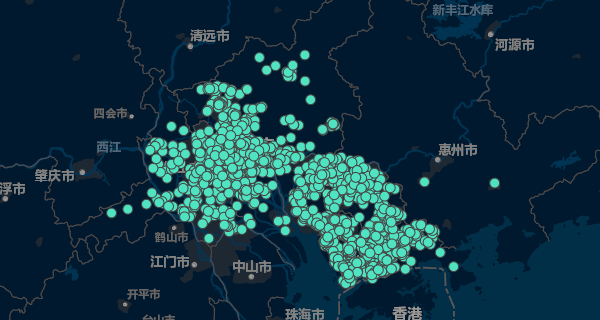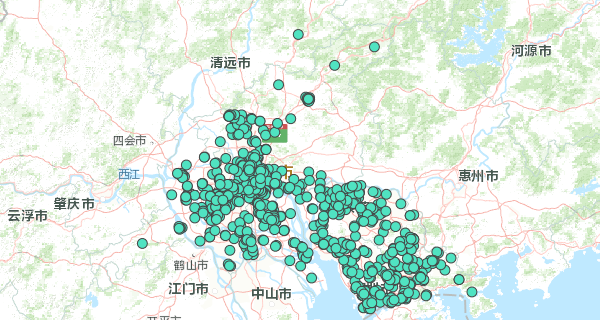从定位之后的图示来看，我们可以不难发现以下两个结论：1.该数据主要收集于广东省的佛山市、广州市、东莞市、深圳市四个城市，后续我们也是针对这四个地区进行分析。2.这些数据中含有特殊值，这里我们将其视为异常值，需要进行特别处理。

##### 5.1.1.2 数据的预处理

从我们所总结的因素出发，由于四个因素所在不同的附录之中，所以我们需要将这两个附录文件进行整合。首先通过附录一中的经纬度数据来确定任务的发布位置，然后由Python中的pandas和numpy两个工具包来对两个附录中的数据进行整合，进而完成该数据的预处理模块。

###### 5.1.1.2.1 附件一数据的预处理

在附件一中，其内含有任务的相关经纬度数据，由于我们在分析任务定价的过程中，将任务发布的地理位置作为主要因素之一，故我们需要确定发布任务的位置与任务所在城市中心之间的距离，以此来具体确认任务定价与任务地理位置的关系。
我们要想计算出任务点与中心位置的距离，则首先需要知道中心位置的经纬度。而由上述的数据定位可知，所有数据整体在佛山、广州、深圳、东莞四个城市中，据资料显示，这是个城市中心的经纬度如下所示：

既然现在已知城市中心以及所有任务点的经纬度信息，我们即可通过以下式子计算出所有任务点与中心之间的距离：

${D}_{ij}=R\ast arccos\left[sin{\phi }_{i}sin{\phi }_{j}+cos{\phi }_{i}cos{\phi }_{j}cos\left({\theta }_{i}-{\theta }_{j}\right)\right]$

Dij D i j $D_{ij}$任务 i i $i$ 与城市中心 $j$$j$ 的距离，单位千米(km)
R R $R$ 地球的半径，单位千米(km)
${\theta }_{i}$$\theta_i$任务 i i $i$ 的经度值
${\phi }_{i}$$\varphi_i$任务 i i $i$ 的纬度值
${\theta }_{j}$$\theta_j$城市 j j $j$ 的经度值
${\phi }_{j}$$\varphi_j$城市 j j $j$ 的纬度值

为此，我们就能由以下相关程序处理附件一中的数据：

def append_length(file_path, jing_value2, wei_value2, file_name, encoding):
length = []
for index1, each_data1 in df1.iterrows():
jing_value1 = each_data1["任务gps经度"]
wei_value1 = each_data1["任务gps 纬度"]
distance = int(6371 * np.arccos(np.sin(wei_value1)*np.sin(wei_value2)+np.cos(wei_value1)*np.cos(wei_value2)*np.cos(jing_value1-jing_value2)))
length.append(distance)
df1["与中心距离"] = length
df1.to_csv(file_name, index=False)
###### 5.1.1.2.2 附件二数据的预处理

在附录二中的会员数据里，我们发现其中的经纬度数据信息在同一字段中，为了分析的方便，我们首先使用pandas将该添加对应的经度和纬度两个字段，相关核心代码如下，完整代码可见附录：

def deal_column(data_path, sheet):
data = pd.ExcelFile(data_path)
df = data.parse(sheet)
locations = df["会员位置(GPS)"]
location_jing = [location.split(" ") for location in locations]
location_wei = [location.split(" ") for location in locations]
df["会员gps经度"] = location_jing
df["会员gps纬度"] = location_wei
df.to_csv(d + "/after_deal.csv", index=False)

处理之后的部分数据如下图所示：###### 5.1.1.2.3 数据异常值的处理

在上述操作中，我们发现附录一和附录二中含有异常值，我们需要针对进行异常值进行特殊处理，否则将会对我们后面的模型造成影响。要想具体确认每个经纬度所对应的具体位置，我们需要借助于GPSspg的相关操作。为此，我们首先将附件一和附件二中的各个位置的经纬度提取出来然后进行具体定位，最后导入到新的文件中,具体定位后的部分数据如下图所示：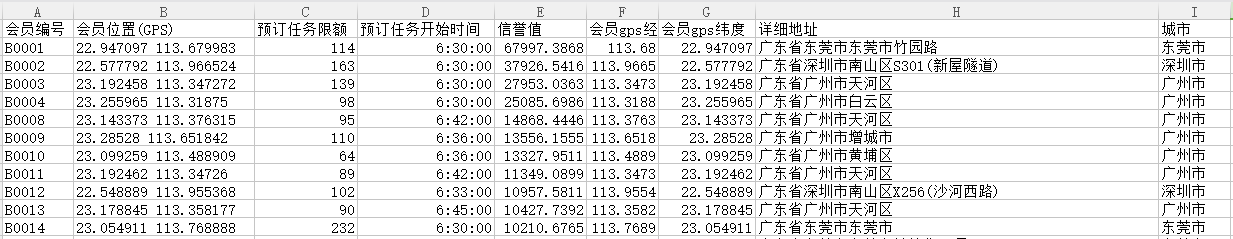通过文件中的数据可以发现，除了上述分析的佛山市、深圳市、广州市、东莞市之外还掺杂了其他地区的数据，例如清远市、中山市、别行政、惠州市等。经统计发现总共含有20个异常数据，它们的编号分别是A0297、A0751、B0005、B0006、B0007、B0022、B0033、B0039、B0048、B0082、B0135、B0136、B0472、B1077、B1585、B1627、B1708、B1727、B1820、B1822。它们的编号和具体数据内容可如图五、图六所示：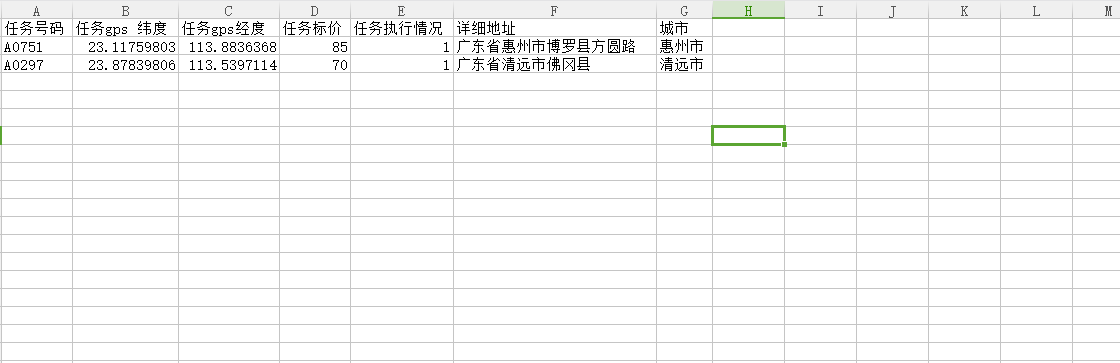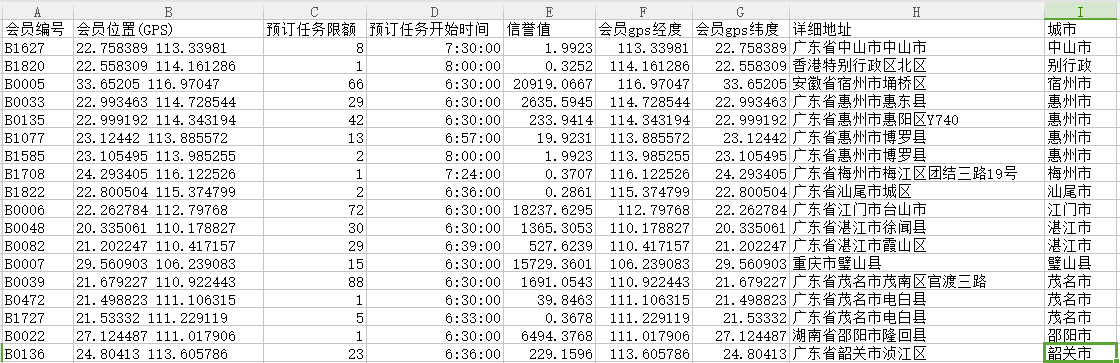为了不影响后面模型的精度，这里我们将这些异常数据进行直接进行删除即可，删除的部分核心代码如下所示：

def delete_data(data_path, sheet):
groups = df.groupby("城市")
error_group = pd.DataFrame(columns=["会员编号","会员位置(GPS)","预订任务限额","预订任务开始时间","信誉值","会员gps经度","会员gps纬度","详细地址","城市"])
true_group = pd.DataFrame(columns=["会员编号","会员位置(GPS)","预订任务限额","预订任务开始时间","信誉值","会员gps经度","会员gps纬度","详细地址","城市"])
for city, group in groups:
if city not in ["佛山市"、"广州市"、"深圳市"、"东莞市"]:
error_group = pd.concat([error_group, group])
else:
true_group = pd.concat([true_group, group])
error_group.to_csv("error_data.csv", index=False)
true_group.to_csv("true_data.csv", index=False)
###### 5.1.1.2.4 数据的整合

由之前对任务定价的分析可知，我们需要将会员数据与任务数据进行整合，从而进一步分析公司在对任务定价的时候是否考虑到会员的相关因素。在此，我们将两个附件的数据进行整合操作的依据是在一定区域范围之内所含有的会员数目，并以此求相关会员信息的均值来对定价进行分析。我们可以通过以下公式进行计算：

${D}_{ik}=R\ast arccos\left[sin{\phi }_{i}\ast sin{\phi }_{k}+cos{\phi }_{i}cos{\phi }_{k}cos\left({\theta }_{i}-{\theta }_{k}\right)\right]$

Dij D i j $D_{ij}$任务 i i $i$ 与会员 $k$$k$ 的距离，单位千米(km)
R R $R$ 地球的半径，单位千米(km)
${\theta }_{i}$$\theta_i$任务 i i $i$ 的经度值
${\phi }_{i}$$\varphi_i$任务 i i $i$ 的纬度值
${\theta }_{k}$$\theta_k$会员 k k $k$ 的经度值
${\phi }_{k}$$\varphi_k$会员 k k $k$ 的纬度值

通过上述距离公式，我们可以借助numpy工具计算每个任务在与会员之间的距离是否在我们规定的范围之内，从而将附件二中的有效数据合并到附件一中，即在附件一中添加四个字段：会员预定任务的限额、会员所在地的集中程度、任务发布时间以及任务发布地的经济状况。其中前三个因素可以在附录一中进行算法提取，而为了分析问题的全面性，这里多考虑到了任务发布地区的经济状况因素，虽然在本题中并未给出该方面的数据，但是我们可以通过查找相关资料进行了解从而进行综合性分析。
通过上述的分析，我们在这规定若任务与会员之间的距离小于 $r$$r$ ，则我们将该会员的数据录入到对应的任务中，即:

r>Dik=Rarccos[sinφisinφk+cosφicosφkcos(θiθk)] r > D i k = R ∗ a r c c o s [ s i n φ i ∗ s i n φ k + c o s φ i c o s φ k c o s ( θ i − θ k ) ]

结合实际情况与各个数据的经纬度的联系，我们在对数据进行整合的时候取 r=500km r = 500 k m $r=500km$ 。由于该部分的核心代码较长，出于对论文可读性的考虑，此部分的代码不在此处进行展示，读者可在附录中进行浏览。整合之后的部分相关数据如下：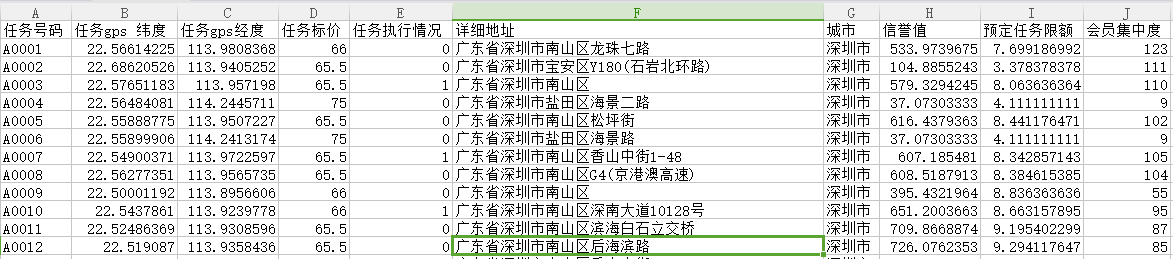##### 5.1.1.3 定价规律可视化及分析
###### 5.1.1.3.1 任务的地点位置对定价的影响分析

为了分析出任务点位置对任务定价的具体影响，我们需要将上述预处理之后的附件一中的数据进行可视化从而得到任务位置对任务定价的影响。为此我们将任务 i i $i$ 定为 ${P}_{i}$$P_i$ ，将任务点与城市中心的距离定为 Dij D i j $D_{ij}$，通过这两个数据便可绘制两者之间的关系图。此处，我们不妨使用散点图将每个数据点在直角坐标系中表示出来，从而分析任务定价随着任务位置之间的大致趋势，进而选择恰当的函数来对数据点进行拟合。除此之外，为了避免城市的不同而对任务价格的影响，我们分别绘制广州、佛山、深圳、东莞的定价位置散点图。基于这种分析，我们使用matplotlib工具绘制出的散点图以及程序的核心代码如下：

def draw_scatter(file_path, color, marker, city, image_path):
p = df["任务标价"]
d = df["与中心距离"]
plt.scatter(d/100, p, s=10, c=color, alpha=0.6, marker=marker)
plt.title("{}任务位置与定价的关系".format(city), fontproperties=font)
plt.xlabel("Distance/x100km")
plt.ylabel("Price")
plt.savefig(image_path)
plt.show()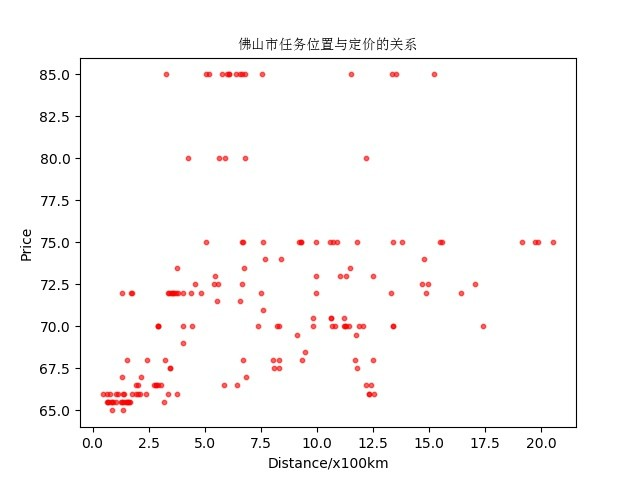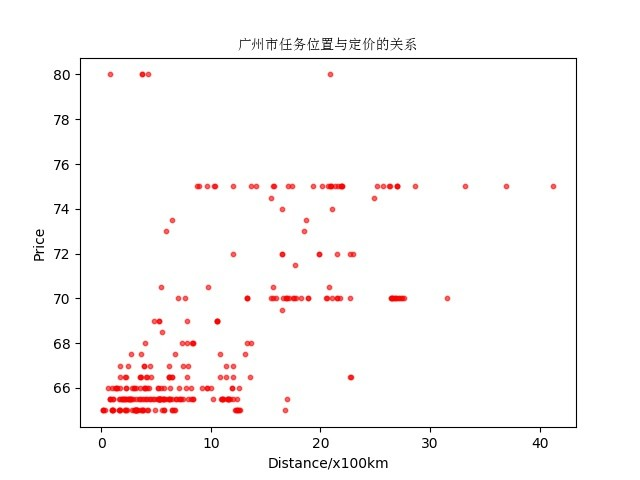从上面的任务定价与任务位置之间的散点图中不难发现，深圳、广州、东莞、佛山整体呈现一种 “上疏下密，右疏左密”的形式。故于此，我们可以得到以下结论：距市中心越进的任务，APP平台对其任务定价相对比较低,定价在65~75之间；而距市中心越远的任务，APP平台对其任务定价相对较高，定价在76~86之间。此外，随着距离的增大，定价的价格增长的幅度整体在逐渐增大。对此，我们将这两种模型近似拟合为一个指数回归模型。
所以，根据上面所述，我们不妨建立一个关于任务点位置对任务定价的影响的模型，为了模型进行的精确性以及拟合效果的良好度，这里我们利用WPSExcel中的散点指数拟合模型进行拟合，且该模型满足以下关系式：

Pi=66.892e35Dij P i = 66.892 ∗ e 3 − 5 D i j

至此，我们已经求解出任务位置与定价的数学模型，而为了确认该模型的拟合程度，我们需要引入拟合优度值 r2 r 2 $r^2$ 来进一步验证该模型。

r2=1mi=0(PiPc)mi=0(PiP¯¯¯¯) r 2 = 1 − ∑ i = 0 m ( P i − P c ) ∑ i = 0 m ( P i − P ¯ )

通过科学计算可得知拟合优度 r2 r 2 $r^2$=0.7317295，该值近似为1可知，该模型的拟合具有比较好的效果。于此，我们可以确认任务的位置与任务的定价存在较大的关系，即距市中心越进的任务，APP平台对其任务定价相对比较低,定价在65~75之间；而距市中心越远的任务，APP平台对其任务定价相对较高，定价在76~86之间。
通过结合现实来讲，存在这种定价规律主要存在以下原因：

• 在距城市中心较近处，具有较大的人口密度且较为发达，完成任务的可能性较大，所以公司为了提高任务的完成率需要增大会员对任务的渴求欲，任务的价格普遍较低。
• 在距城市中心较远处，地理位置比较偏僻且周围环境相对比较恶劣，完成任务的可能性比较小，所以公司进行提高任务定价的方式来诱导会员完成任务。

随后，通过我们上面所描述，任务点的经纬度与城市中心满足以下关系：

Dij=Rarccos[sinφisinφj+cosφicosφjcos(θiθj)] D i j = R ∗ a r c c o s [ s i n φ i s i n φ j + c o s φ i c o s φ j c o s ( θ i − θ j ) ]

所以，最终我们将任务的定价与任务的经纬度的关系模型定义如下：

Pi=66.892e35Rarccos[sinφisinφj+cosφicosφjcos(θiθj)] P i = 66.892 ∗ e 3 − 5 R ∗ a r c c o s [ s i n φ i s i n φ j + c o s φ i c o s φ j c o s ( θ i − θ j ) ]

###### 5.1.1.3.2 任务发布地的经济状况

除了任务距城市中心的距离对任务的定价造成影响之外，城市的经济状况也会对其产生一定的影响。而为分析此种影响，我们需要对以上所述的四个城市的经济状况有所了解。据资料显示，四个城市的GDP以及变化率如下所示：

之后，我们通过matplotlib来绘制四个城市的任务平均价格关系图，相关程序及图示结果如下所示：

def get_mean_price(file_path):
p = df["任务标价"]
mean_price = np.mean(p)
return mean_price

if __name__ == "__main__":
files = [parent_path + "/newdata/cleaneddata/final_data1_sz.csv", parent_path + "/newdata/cleaneddata/final_data1_gz.csv", parent_path + "/newdata/cleaneddata/final_data1_fs.csv", parent_path + "/newdata/cleaneddata/final_data1_dg.csv"]
price_ls = [get_mean_price(each_file) for each_file in files]
plt.barh(np.arange(4), price_ls, height=0.7, color="lightskyblue")
plt.xlabel("Price")
plt.ylabel("Cities")
plt.title("城市与任务定价的关系", fontproperties=font)
plt.yticks(np.arange(4), ["深圳", "广州", "佛山", "东莞"], rotation=45, size=8)
for x, value in enumerate(price_ls):
plt.text(y=x, x=value, s="{}".format(value), ha="center", )
plt.savefig(parent_path + "/images/city_price.jpg")
plt.show()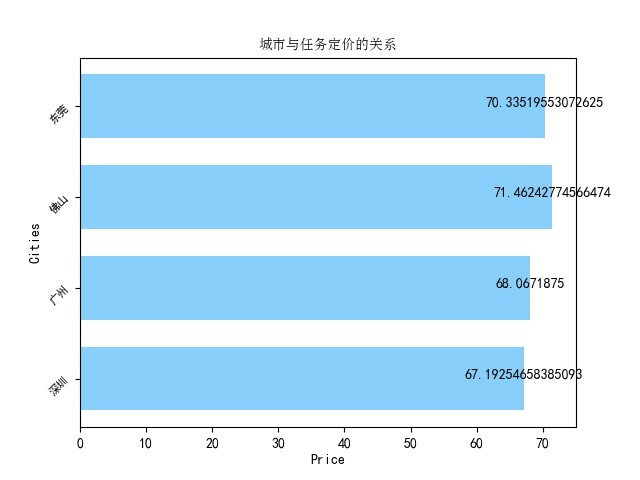从图中我们可以了解到，东莞与佛山的任务定价相对较高，而广州和深圳的任务定价相对较低。这与上述各个城市的经济状况正好呈现一种相反的趋势，这种趋势理解为城市的经济状况与任务定价成负相关。

###### 5.1.1.3.3 会员所在地的集中程度

从实际出发，公司在给任务进行定价的时候，一般会考虑到一定区域内会员的分布密度，且在分布密度较小的区域内公司会提高任务的定价，以此来吸引更多的会员，从而提高APP平台访问的用户量，进而使公司的利益最大化。为此，我们同样需要由整合之后的数据来分析会员集中度对任务定价的影响，最终实现的效果图如下所示：由上图我们可以看出，结果与我们料想的如出一辙。会员的集中度与任务的定价整体存在某种负相关关系。

#### 5.1.2 任务未完成原因分析

##### 5.1.2.1 任务未完成数据处理

在进行分析任务未完成原因分析时，首先我们需要对任务未完成的数据进行处理，该处理部分我们主要通过任务未完成任务数据的提取来进行。相关处理核心代码如下：

def split_yes_no(file_name1, file_name2):
for status, group in df.groupby("任务执行情况"):
if status == 0:
group.to_csv(file_name1, index=False)
else:
group.to_csv(file_name2, index=False)

在分解之后，我们发现未完成的任务数据有313组，后面我们将针对这313组的数据进行原因分析。

##### 5.1.2.2 任务的定价过低

首先，我们不难发现，若公司对一组任务的定价过低，则定价很大可能不足以引起会员的接单欲望。所以，任务的定价过低很有可能是造成任务未完成的原因之一。
通过对数据的观察我们可以发现，任务的定价皆在65~85之间。为此，我们将任务分为四个定价区间，即65~69、70~74、75~79、80~85四个数据区域。通过此分析，我们可以绘制数据的散点图以及他们的相关统计图，相关核心代码以及结果图示如下：

def draw_no_scatter():
plt.scatter(np.arange(313), df["任务标价"], s=20, c="red", alpha=0.6, marker="*")
plt.title("任务未完成数据的价格分布")
plt.xlabel("Mission")
plt.ylabel("Price")
plt.savefig(parent_path + "/images/no_price.jpg")
plt.show()
def draw_no_bar():
df1 = df[(65 <= df["任务标价"]) & (df["任务标价"] < 70)]
df2 = df[(70 <= df["任务标价"]) & (df["任务标价"] < 75)]
df3 = df[(75 <= df["任务标价"]) & (df["任务标价"] < 80)]
df4 = df[(80 <= df["任务标价"]) & (df["任务标价"] < 85)]
data_len = [len(df1), len(df2), len(df3), len(df4)]
plt.barh(np.arange(4), data_len, height=0.6, alpha=0.7, color="yellowgreen")
plt.ylabel("Price Region")
plt.xlabel("Num")
plt.title("未完成价格条形图")
plt.yticks(np.arange(4), ["65-69", "70-74", "75-79", "80-85"], rotation=45, size=13)
for x, value in enumerate(data_len):
plt.text(y=x, x=value, s="{}".format(value), ha="center")
plt.savefig(parent_path+"/images/price_bar.jpg")
plt.show()

plt.legend(["65-69", "70-74", "75-79", "80-85"], loc="best")
plt.title("未完成价格饼图")
plt.savefig(parent_path+"/images/price_pie.jpg")
plt.show()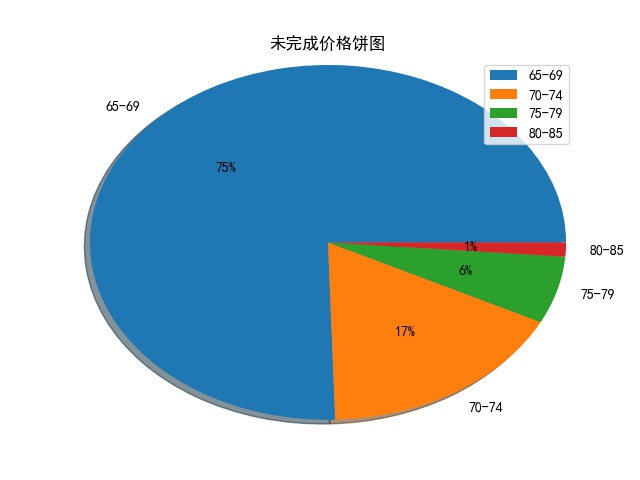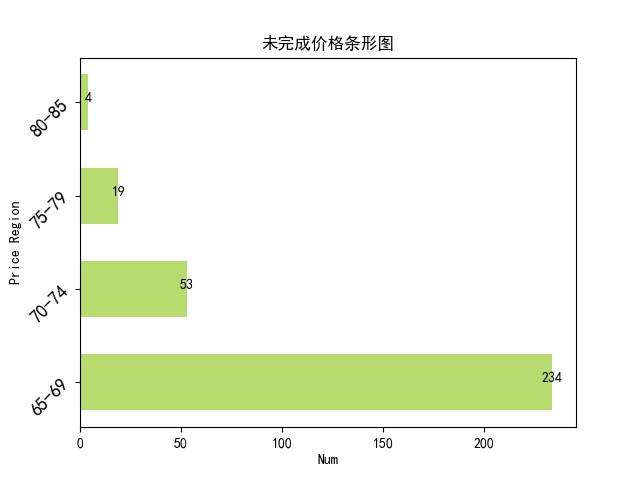通过以上图的显示，我们在散点图中可以明显的看出任务定价在65-75的区间内明显占据绝大部分。从条形图和饼图中我们也能看出其所占比例之大。为此，我们可以确定之前的结论，即公司对任务定价过低的时候，不足以勾起会员完成任务的欲望，也就是说会员完成任务的可能性极小。

##### 5.1.2.3 任务发布位置较远

在原因一的分析中，除了任务定价在65-75之间之外，还有23组的任务的定价相对来说是比较高的。那么既然如此，会员未完成任务的原因是什么呢？为此，我们在这需要分析任务是否离城市中心的距离较远，从而导致会员完成任务的难度增大。

#### 5.1.3 问题一总结

通过以上模块的分析，我们得出公司对任务定价主要从任务点位置、任务发布地的经济状况、会员预定任务限额、信誉值、集中度所决定。而任务未完成的原因主要是任务的定价较低以及任务较远导致。

### 5.2 多维度定价方案对项目的效益——问题二

“拍照赚钱”APP的任务定价是一种任务发布者（APP平台）与任务接受者（平台会员）之间所进行的互利模式，PP平台希望在成本一定的情况下使得任务的完成率尽可能的提高，而APP会员则需要在满足某种条件要求下追求收益尽可能大。在问题二中，要求为项目设计新的任务定价方案，则需要考虑以下几个方面：

• 该项目的总收益由两个因素决定，一是任务的实际定价，二是任务的实际完成情况。
• 站在公司的角度考虑，任务的定价是由平台根据相关数据而定的，而任务的定价在问题一中我们已做分析，影响任务定价的因素有该区域会员的信誉值、会员预定任务限额、会员与任务之间的距离、该区域的经济发展状况以及会员的集中密度。
• 站在会员的角度分析，任务的完成率由会员根据实际情况而定，而对任务完成率的影响因素主要有，任务的价格、会员与任务之间的距离以及该任务的竞争程度。

除此之外，从实际出发，一份任务理论上可以分配给多个使其相互竞争，而一个会员亦可接受多份任务，这两者在初始阶段是一种“多对多”的关系。而最终的情况是一个任务只能分配给一个会员，而一个会员依然可以接受多份任务，这是一种“多对一”的关系。通过以上对该问题的分析，我们可以构建项目-会员-任务之间的关系图：通过上述的分析，对此本文主要从以下四个步骤进行解决该问题：

Step1. 确定任务-会员空间分配算法
Step2. 由问题一分析出的多因素对任务定价造成的影响，建立出多维度空间任务定价模型。
Step3. 对任务完成度进行分析并构建会员抉择概率模型。
Step4. 通过上述模型的构建最终确定能够体现出新方案效果的项目总收益模型。
Step5. 使用Matlab、Python、Lingo三种编程语言来对模型进行求解并总结新方案的优势。

#### 5.2.1 模型构建

##### 5.2.2.1 任务-会员空间分配

首先，我们需要一个算法来对任务、会员之间进行合理的分配，以便后续模型的构建。出于体现出全国大学生数学建模竞赛的创新性，以下给出该任务-会员空间分配算法的实现流程图：用 xi x i $x_i$ 表示第 i(i=1,2,,n) i ( i = 1 , 2 , ⋯ , n ) $i(i=1,2,\cdots,n)$ 个任务， yj y j $y_j$ 表示第 k(k=1,2,,m) k ( k = 1 , 2 , ⋯ , m ) $k(k=1,2,\cdots,m)$ 个会员。以任务为节点，任务与每位会员之间的距离为对应的权重，为此构建该城市的任务-会员距离矩阵 L(δ)(δ=1,2,3,4) L ( δ ) ( δ = 1 , 2 , 3 , 4 ) $L^{(\delta)} (\delta=1,2,3,4)$，如下所示：

L(δ)=(D(δ)ik)n×m=D(δ)11D(δ)21D(δ)31D(δ)n1D(δ)12D(δ)22D(δ)32D(δ)n2D(δ)13D(δ)23D(δ)33D(δ)n3D(δ)1mD(δ)2mD(δ)3mD(δ)nm(5)(6) (5) L ( δ ) = ( D i k ( δ ) ) n × m (6) = ( D 11 ( δ ) D 12 ( δ ) D 13 ( δ ) ⋯ D 1 m ( δ ) D 21 ( δ ) D 22 ( δ ) D 23 ( δ ) ⋯ D 2 m ( δ ) D 31 ( δ ) D 32 ( δ ) D 33 ( δ ) ⋯ D 3 m ( δ ) ⋮ ⋮ ⋮ ⋱ ⋮ D n 1 ( δ ) D n 2 ( δ ) D n 3 ( δ ) ⋯ D n m ( δ ) )

D(δ)ik=Rarccos[sinφisinφk+cosφicosφkcos(θiθk)] D i k ( δ ) = R ∗ a r c c o s [ s i n φ i ∗ s i n φ k + c o s φ i c o s φ k c o s ( θ i − θ k ) ]

##### 5.2.2.2 多维度空间任务定价模型

通过问题一的分析，我们已经知道了多因素对任务定价产生的影响规律，主要体现在以下多个方面：

• 在以某个任务为中心的一定区域内，若该区域中的会员信誉值较小时，则该任务的定价普遍较低。
• 随着会员离任务的距离逐渐增大，则任务的定价普遍按照一定的波动规律有所提高。
• 若城市的经济状况较为发达，则该城市的任务定价相对较低。
• 如若会员的集中密度逐渐增大，则任务的定价按照一定趋势有所下降。

在上一模块中，我们已经通过任务-会员空间分配模型分别对任务分配了相对应的会员量，并将其距离数据存储于矩阵 Lδ L δ $L_\delta$ 中，但为了避免影响其他因素之间复杂的相关性以及给模型求解带来一定的难度，我们首先需要使用 Zscore Z − s c o r e [ 1 ] $Z-score^{}$模型对矩阵中数据进行归一化处理：

D(δ)ik=D(δ)ikμσ D i k ( δ ) ∗ = D i k ( δ ) − μ σ

σ=1n×mi=1nk=1m(D(δ)ikμ)2 σ = 1 n × m ∑ i = 1 n ∑ k = 1 m ( D i k ( δ ) − μ ) 2

μ=1n×mi=1nk=1mD(δ)ik,  μ = 1 n × m ∑ i = 1 n ∑ k = 1 m D i k ( δ ) ,

L(δ)=(D(δ)ik)n×m L ( δ ) ∗ = ( D i k ( δ ) ∗ ) n × m

同理，我们可以对矩阵中所对应会员的信誉值做归一化处理。通过上述分析，为此，我们可以构建多维度空间任务定价模型：

Pi=KE(δ)(K1Ci¯¯¯¯¯ρi)a+(K2Di¯¯¯¯¯¯ρi)aa+ξ P i = K E ( δ ) ( K 1 C i ¯ ρ i ) a + ( K 2 D i ¯ ρ i ) a a + ξ

Ci¯¯¯¯¯=1mk=1mck, Di¯¯¯¯¯¯=1mk=1mDik C i ¯ = 1 m ∑ k = 1 m c k ,   D i ¯ = 1 m ∑ k = 1 m D i k

其中， Ci¯¯¯¯¯ C i ¯ $\overline{C_i}$ 表示该任务点所在领域内会员信誉期望值， Di¯¯¯¯¯¯ D i ¯ $\overline{D_i}$ 表示任务点所在领域内会员位置期望值， E(δ) E ( δ ) $E^{(\delta)}$表示任务所在城市的经济系数， ρi ρ i $\rho_i$ 表示任务所在领域内会员密度值， K,K1,K2 K , K 1 , K 2 $K,K_1,K_2$ 表示价格与多维度因素之间的比例系数， ξ ξ $\xi$ 表示该方案的容错误差值。

同时，我们可以通过上述四个城市的GDP（国内生产总值）来确定该模型的经济系数 E(δ) E ( δ ) $E^{(\delta)}$

E(δ) E ( δ ) $E^{(\delta)}$1.9492601.9610940.8003920.627506

最终，确定的多维度空间任务定价模型如下：

Pi=KE(δ)(K11mρik=1mck)a+(K21mρik=1mDik)aa+ξ P i = K E ( δ ) ( K 1 1 m ρ i ∑ k = 1 m c k ) a + ( K 2 1 m ρ i ∑ k = 1 m D i k ) a a + ξ

##### 5.2.2.3 会员抉择概率模型

同样，在上述的分析中，我们已经把影响任务完成度的因素归结于任务的定价、任务位置、任务竞争度。且这三种因素的影响如下：

• 若某一任务的定价较高时，任务对会员的吸引度较大，该任务被完成的概率也随之增大。
• 从实际出发，若任务离会员越远，任务对会员的吸引度越小，该任务被完成的概率也随之减小。
• 若一任务的会员竞争度较大，即该会员被较多的会员相中，相应的任务被完成的概率较高。

首先，任务完成与否是由会员对任务所能获得期待的效益决定，当该效益值达到一定的阈值时，我们可以认为该任务被领域内的会员完成的概率较高，对此，我们应该建立该任务-会员吸引度模型：

A=λPiQiDi¯¯¯¯¯¯ A = λ P i Q i D i ¯

其中， Qi Q i $Q_i$ 表示任务的竞争度，在这里我们可以将上述任务-会员矩阵中每行的会员数。根据实际情况，我们认为若一个任务的定价小于70，归一化后的距离大于0.8，被相中的会员数小于3，我们任务该任务完成的概率为0，其所对应的任务对会员的吸引度为 A110 A 1 ≤ 10 $A_1\leq10$；同样若某个任务的定价大于80，归一化后的距离小于0.2，被相中的会员数大于8，我们认为该任务被完成的概率为1，其所对应的任务吸引度为 A190 A 1 ≥ 90 $A_1\geq90$。根据上述信息，我们可以确定 λ λ $\lambda$ 的值：

λ=12(A1D1i¯¯¯¯¯¯P1iQ1i+A2D2i¯¯¯¯¯¯P2iQ2i) λ = 1 2 ( A 1 D i 1 ¯ P i 1 Q i 1 + A 2 D i 2 ¯ P i 2 Q i 2 )

经计算， λ=0.042634 λ = 0.042634 $\lambda=0.042634$。对此，我们可以确定任务-会员吸引度模型最终为：

A=A1,0.042634PiQiDi¯¯¯¯¯¯,A2,Pi<70,Qi<3,Di¯¯¯¯¯¯>0.8othersPi>80,Qi>8,Di¯¯¯¯¯¯<0.2 A = { A 1 , P i < 70 , Q i < 3 , D i ¯ > 0.8 0.042634 P i Q i D i ¯ , o t h e r s A 2 , P i > 80 , Q i > 8 , D i ¯ < 0.2

根据任务-会员吸引度模型，我们可以建立出会员抉择概率模型：

Zi=0,0.042634PiQi100×Di¯¯¯¯¯¯,1,0.042634PiQiDi¯¯¯¯¯¯<10100.042634PiQiDi¯¯¯¯¯¯<900.042634PiQiDi¯¯¯¯¯¯90 Z i = { 0 , 0.042634 P i Q i D i ¯ < 10 0.042634 P i Q i 100 × D i ¯ , 10 ≤ 0.042634 P i Q i D i ¯ < 90 1 , 0.042634 P i Q i D i ¯ ≥ 90

Pi=KE(δ)(K11mρik=1mck)a+(K21mρik=1mDik)aa+ξ 其 中 ， P i = K E ( δ ) ( K 1 1 m ρ i ∑ k = 1 m c k ) a + ( K 2 1 m ρ i ∑ k = 1 m D i k ) a a + ξ

##### 5.2.2.4 项目总收益模型

我们已经分析得到项目的总收益是由任务定价以及任务的完成情况而定，而在上面我们确定了任务多维度空间任务定价模型和会员抉择概率模型，由此，我们可以构建出项目总收益模型，以分析出新方案的实施效果，项目的总收益根据会员抉择概率模型可分为三个部分：不可能收益、绝对收益、概率收益。其一般式如下：

W(δ)=i=1nPiZi=W1+W2+W3=0+1lPi+i=lnPiZi(7)(8) (7) W ( δ ) = ∑ i = 1 n P i Z i = W 1 + W 2 + W 3 (8) = 0 + ∑ 1 l P i + ∑ i = l n P i Z i

之后，将上述的任务多维度空间定价模型和会员抉择概率模型代入其中，可最终确定项目总收益模型：

W(δ)=i=1l(KE(δ)(K11mρik=1mck)a+(K21mρik=1mDik)aa+ξ)+i=ln0.042634QiDi¯¯¯¯¯¯(KE(δ)(K11mρik=1mck)a+(K21mρik=1mDik)aa+ξ)2(9)(10) (9) W ( δ ) = ∑ i = 1 l ( K E ( δ ) ( K 1 1 m ρ i ∑ k = 1 m c k ) a + ( K 2 1 m ρ i ∑ k = 1 m D i k ) a a + ξ ) (10) + ∑ i = l n 0.042634 Q i D i ¯ ( K E ( δ ) ( K 1 1 m ρ i ∑ k = 1 m c k ) a + ( K 2 1 m ρ i ∑ k = 1 m D i k ) a a + ξ ) 2

#### 5.2.2 模型求解

首先，我们需要通过上述的任务-会员分配算法对任务、会员之间进行分配，部分程序代码和分配结果如下：

[a, b] = size(data1_dg_jing);
[c, d] = size(data2_dg_jing);
mt_data = zeros(a,c);
for i = 1:a
for j = 1:c
mt_data(i,j) = 6371*acos(sin(data1_dg_wei(i))*sin(data2_dg_wei(j))+cos(data1_dg_wei(i))*cos(data2_dg_wei(j))*cos(data1_dg_jing(i)-data2_dg_jing(j)));
end
end
normalized_data = zscore(mt_data);
for i = 1:a
for j = 1:c
if normalized_data(i,j) > 0
normalized_data(i,j) = 0;
end
end
end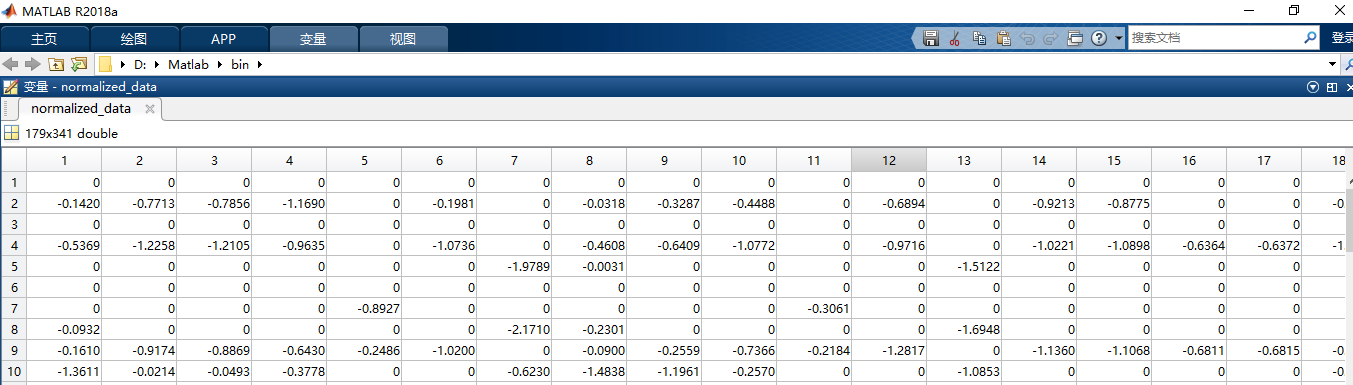通过上述对任务-会员进行分配之后，我们可以使用Matlab进行网格搜索来确定各个需要的系数值，最终我们可以将多维度空间任务定价模型定义如下：

Pi=0.6359E(δ)(3.83741mρik=1mck)2+(5.69131mρik=1mDik)2±5 P i = 0.6359 E ( δ ) ( 3.8374 1 m ρ i ∑ k = 1 m c k ) 2 + ( 5.6913 1 m ρ i ∑ k = 1 m D i k ) 2 ± 5

E(δ)=1.949260,1.961094,0.800392,0.627506 E ( δ ) = 1.949260 , 1.961094 , 0.800392 , 0.627506

而会员抉择概率模型我们在上面已经进行分析，其与任务定价、距离、竞争有关，对此我们同样可以确定最终的会员抉择概率模型并进一步求解项目总收益模型：

W(δ)=i=1l(0.6359E(δ)(3.8374Ci¯¯¯¯¯ρi)2+(5.6913Di¯¯¯¯¯¯ρi)2±5)+i=ln0.042634QiDi¯¯¯¯¯¯(0.6359E(δ)(3.8374Ci¯¯¯¯¯ρi)2+(5.6913Di¯¯¯¯¯¯ρi±5)2(11)(12) (11) W ( δ ) = ∑ i = 1 l ( 0.6359 E ( δ ) ( 3.8374 C i ¯ ρ i ) 2 + ( 5.6913 D i ¯ ρ i ) 2 ± 5 ) (12) + ∑ i = l n 0.042634 Q i D i ¯ ( 0.6359 E ( δ ) ( 3.8374 C i ¯ ρ i ) 2 + ( 5.6913 D i ¯ ρ i ± 5 ) 2

其对应的优化模型如下：

max δ=14W(δ)s.t.min(C1,C2,,Ci)Ci¯¯¯¯¯max(C1,C2,,Ci)min(D1,D2,,Di)Di¯¯¯¯¯¯max(D1,D2,,Di)i=1,2,,n m a x   ∑ δ = 1 4 W ( δ ) s . t . { m i n ( C 1 , C 2 , ⋯ , C i ) ≤ C i ¯ ≤ m a x ( C 1 , C 2 , ⋯ , C i ) m i n ( D 1 , D 2 , ⋯ , D i ) ≤ D i ¯ ≤ m a x ( D 1 , D 2 , ⋯ , D i ) i = 1 , 2 , ⋯ , n

对于该最优化模型，我们使用Lingo对其进行求解，之后使用新方案对附件一中的任务数据进行处理，最终获得的结果部分如下所示：#### 5.2.3 模型求解分析与问题总结

通过上述的模型构建以及模型求解，我们制订了任务定价的新方案，而且对于新方案，其优势可以由以下几个方面总结：

• 在任务完成方面，原方案中含有313个未完成任务，而在使用新方案之后任务未完成数减少到213个，其任务完成率从 62.425% 62.425 % $62.425\%$ 提高到了 74.430% 74.430 % $74.430\%$，新方案对任务完成情况的优化程度为 12.005% 12.005 % $12.005\%$

• 在项目实际收益方面，原方案的实际收益为 36291 36291 $36291$，新方案的实际收益为 42964 42964 $42964$，收益率提高了 18.3875% 18.3875 % $18.3875\%$

• 在项目的理想收益方面，原方案该项目的理想收益为 57552.5 57552.5 $57552.5$，而在使用了新方案之后，该项目的理想收益是 54319 54319 $54319$

综上所述，我们所制订的新方案一方面能够让APP平台的收益有个较大的提高，另一方面同时能够使会员的收入有所增多，即在APP平台和会员之间实现了双赢的效果，在双方获利的同时还有利于之间的长期合作。

### 5.3 基于打包方案的优化——问题三

在本问中，提到实际情况下多个任务可能会因为位置比较集中而导致用户会争相选择，为此我们需要采用一种打包方式来对原方案进行优化。在采用任务打包方案之后之后虽然能够提高平台的任务发布效率，但是我们必须首先解决以下两个问题：

• 制订一个合理的方案来对任务进行打包从而进一步提高任务的完成率，即每个打包任务簇中的任务数需要确定。

• 任务簇中的任务数的确定由哪些因素决定

#### 5.3.1 基于打包方案的优化模型建立及求解

在问题二的多维度空间任务定价模型、会员抉择概率模型、项目收益模型中，他们之间是一种环环相扣的关系。而打包任务定价模型是建立在多位度空间任务定价模型的基础上的，所以我们优化的主要模型是多维度空间任务定价模型，继而改变打包任务的定价来体现出优化方案的优势。

1） 会员信誉值及预定任务限额分析

结合实际情况，从APP平台的角度分析，打包任务的任务数是由该打包区域中的所有会员的信誉值和预定任务的限额所决定。为此，我们需要进一步分析会员信誉值和预定任务限额的分布规律。
要想了解会员预定任务的限额以及信誉值对任务定价的影响，我们首先需要用matplotlib绘图工具分别绘制以下两种因素对定价的影响：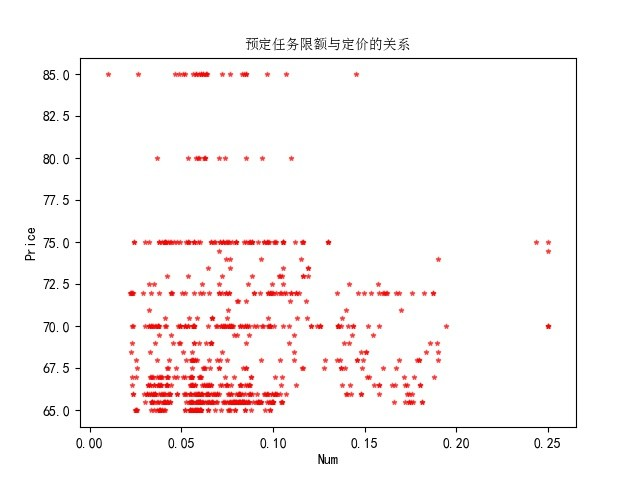由上述图示我们可以知道，数据点主要集中在图示的左下端部分，即在会员信誉值及预定任务额较低的时候，任务的定价普遍较低；反之，任务定价相对较高。
为了避免影响其他因素之间复杂的相关性以及给模型求解带来一定的难度，我们首先需要对会员信誉值 Qk Q k $Q_k$ 和预定任务限额 Mk M k $M_k$ 进行标准化处理：

Qk=Qkμσ Q k ∗ = Q k − μ σ

σ=1mk=1m(Qkμ)2,  μ=1mk=1mQk σ = 1 m ∑ k = 1 m ( Q k − μ ) 2 ,     μ = 1 m ∑ k = 1 m Q k

通过统计我们发现，会员信誉值和预定任务限额呈现一种“中间高，两头低”的特征。对此，我们认为会员的信誉值和预定任务限额满足二维高斯分布  [ 2 ] $^{}$，其概率密度函数如下所示：

f(Qk,Mk)=12πσ1σ21ρ2e12(1ρ2)((Qkμ1)2σ21+2ρ(Qkμ1)(Mkμ2)σ1σ2+(Mkμ2)2σ22) f ( Q k ∗ , M k ∗ ) = 1 2 π σ 1 σ 2 1 − ρ 2 e − 1 2 ( 1 − ρ 2 ) ( ( Q k ∗ − μ 1 ) 2 σ 1 2 + 2 ρ ( Q k ∗ − μ 1 ) ( M k ∗ − μ 2 ) σ 1 σ 2 + ( M k ∗ − μ 2 ) 2 σ 2 2 )

其中， μ1,μ2,σ1,σ2,ρ μ 1 , μ 2 , σ 1 , σ 2 , ρ $\mu_1,\mu_2,\sigma_1,\sigma_2,\rho$ 都是常数，我们把该分布记作 N(μ1,μ2,σ1,σ2,ρ) N ( μ 1 , μ 2 , σ 1 , σ 2 , ρ ) $N(\mu_1,\mu_2,\sigma_1,\sigma_2,\rho)$

2）打包方案任务定价模型

在确定了会员信誉值和预定任务限额的分布以及对任务定价影响规律之后，我们需要建立打包方案的任务定价模型。当概率 f(Qk,Mk) f ( Q k ∗ , M k ∗ ) $f(Q_k^*,M_k^*)$ 达到一定值时，我们可以认为该会员对任务的影响较大，即某任务簇中的任务数较多，入包的可能性较大，对此，我们先建立一个任务入包威胁矩阵模型

J=(Ri)a×b J = ( R i ) a × b

其中， a a $a$ 表示最终打包的任务簇数，$b$$b$ 表示附件二中的任务数，而 Ri R i $R_i$ 表示该任务簇中是否包含了 Ri(i=1,2,,n) R i ( i = 1 , 2 , ⋯ , n ) $R_i(i=1,2,\cdots,n)$，在这里为0-1值，具体表示如下：

Ri={0,1,1nni=1fi(Qk,Mk)>0.8others R i = { 0 , 1 n ∑ i = 1 n f i ( Q k ∗ , M k ∗ ) > 0.8 1 , o t h e r s

最终，确定的打包任务定价模型以及完成概率模型为：

Pi=l(Ri)KaE(δ)(K11mρik=1mck)2+(K21mρik=1mDik)2+ξ P i = l ( R i ) K a E ( δ ) ( K 1 1 m ρ i ∑ k = 1 m c k ) 2 + ( K 2 1 m ρ i ∑ k = 1 m D i k ) 2 + ξ

Zi=0,0.042634Pi(Qi+Mi)f(Qi,Mi)(a+b)Di¯¯¯¯¯¯,1,0.042634Pi(Qi+Mi)f(Qi,Mi)(a+b)Di¯¯¯¯¯¯<10others0.042634Pi(Qi+Mi)f(Qi,Mi)(a+b)Di¯¯¯¯¯¯90 Z i = { 0 , 0.042634 P i ( Q i + M i ) f ( Q i , M i ) ( a + b ) D i ¯ < 10 0.042634 P i ( Q i + M i ) f ( Q i , M i ) ( a + b ) D i ¯ , o t h e r s 1 , 0.042634 P i ( Q i + M i ) f ( Q i , M i ) ( a + b ) D i ¯ ≥ 90

#### 5.3.2 问题总结及分析

同问题二，我们最终可以求解出打包方案的实际收益总值为 45163 45163 $45163$，未完成的任务数为 174 174 $174$ 个，项目的理想收益为 51974 51974 $51974$。相对问题二中未使用打包方案而言，任务完成率提高了 9.341% 9.341 % $9.341\%$，收益率提高了 13.641% 13.641 % $13.641\%$
通过上述结果显示，我们可以得出结论：制订打包方案在一定程度上不仅能够提高APP平台任务的效率，同时还能够提高自身的效益。

## 参考文献

潘斌，于晶贤，衣娜. 数学建模教程[M]. 化学工业出版社，2017.
韩中庚. 数学建模方法及其应用[M]. 高等教育出版社，2017.

## 附录

### 附录一：数据处理程序文件

import pandas as pd
import numpy as np
from matplotlib import pyplot as plt
import os
import numpy as np

d = os.path.dirname(__file__)
parent_path = os.path.dirname(d)
data_path1 = parent_path + "/problems/附件一：已结束项目任务数据.xls"
data_path2 = parent_path + "/problems/附件二：会员信息数据.xls"
data_path3 = parent_path + "/newdata/after_deal.csv"
data_path4 = parent_path + "/newdata/data1.csv"
data_path5 = parent_path + "/newdata/data2.csv"
data_path6 = parent_path + "/newdata/true_data1.csv"
data_path7 = parent_path + "/newdata/true_data2.csv"

def deal_column(data_path, sheet):
data = pd.ExcelFile(data_path)
df = data.parse(sheet)
locations = df["会员位置(GPS)"]
location_jing = [location.split(" ") for location in locations]
location_wei = [location.split(" ") for location in locations]
df["会员gps经度"] = location_jing
df["会员gps纬度"] = location_wei
df.to_csv(d + "/after_deal.csv", index=False)

def create_city1(data_path, path_name):
cities = [each["详细地址"][3:6] for index, each in df.iterrows()]
df["城市"] = cities
df.to_csv(path_name, index=False)

def create_city2(data_path, path_name):
cities = [each["详细地址"][3:6] for index, each in df.iterrows()]
df["城市"] = cities
df.to_csv(path_name, index=False)

def delete_data1(data_path, error_file, true_file):
groups = df.groupby("城市")
error_group = pd.DataFrame(columns=["任务号码","任务gps 纬度","任务gps经度","任务标价","任务执行情况","详细地址","城市"])
true_group = pd.DataFrame(columns=["任务号码","任务gps 纬度","任务gps经度","任务标价","任务执行情况","详细地址","城市"])
for city, group in groups:
if city not in ["佛山市","广州市","深圳市","东莞市"]:
error_group = pd.concat([error_group, group])
else:
true_group = pd.concat([true_group, group])
error_group.to_csv(error_file, index=False)
true_group.to_csv(true_file, index=False)

def delete_data2(data_path, error_file, true_file):
groups = df.groupby("城市")
error_group = pd.DataFrame(columns=["会员编号","会员位置(GPS)","预订任务限额","预订任务开始时间","信誉值","会员gps经度","会员gps纬度","详细地址","城市"])
true_group = pd.DataFrame(columns=["会员编号","会员位置(GPS)","预订任务限额","预订任务开始时间","信誉值","会员gps经度","会员gps纬度","详细地址","城市"])
for city, group in groups:
if city not in ["佛山市","广州市","深圳市","东莞市"]:
error_group = pd.concat([error_group, group])
else:
true_group = pd.concat([true_group, group])
error_group.to_csv(error_file, index=False)
true_group.to_csv(true_file, index=False)

# delete_data1(data_path4, parent_path+"/newdata/error_data1.csv", parent_path+"/newdata/true_data1.csv")

def append_length(file_path, jing_value2, wei_value2, file_name, encoding):
length = []
for index1, each_data1 in df1.iterrows():
jing_value1 = each_data1["任务gps经度"]
wei_value1 = each_data1["任务gps 纬度"]
distance = int(6371 * np.arccos(np.sin(wei_value1)*np.sin(wei_value2)+np.cos(wei_value1)*np.cos(wei_value2)*np.cos(jing_value1-jing_value2)))
length.append(distance)
df1["与中心距离"] = length
df1.to_csv(file_name, index=False)

# append_length(parent_path + "/newdata/cleaneddata/data1_gz.csv", 113.27, 23.13, parent_path + "/newdata/cleaneddata/final_data1_gz.csv", "gbk")
# append_length(parent_path + "/newdata/cleaneddata/data1_fs.csv", 113.12, 23.02, parent_path + "/newdata/cleaneddata/final_data1_fs.csv", "gbk")
# append_length(parent_path + "/newdata/cleaneddata/data1_dg.csv", 113.75, 23.05, parent_path + "/newdata/cleaneddata/final_data1_dg.csv", "gbk")
# append_length(parent_path + "/newdata/cleaneddata/data1_sz.csv", 114.05, 22.55, parent_path + "/newdata/cleaneddata/final_data1_sz.csv", "gbk")

def split_data(file_path, file1, file2, file3,file4, encoding):
groups = df.groupby("城市")
for city, group in groups:
if city == "广州市":
group.to_csv(file1, index=False)
if city == "佛山市":
group.to_csv(file2, index=False)
if city == "深圳市":
group.to_csv(file3, index=False)
if city == "东莞市":
group.to_csv(file4, index=False)

#split_data(data_path6, parent_path + "/newdata/cleaneddata/data1_gz.csv", parent_path + "/newdata/cleaneddata/data1_fs.csv", parent_path + "/newdata/cleaneddata/data1_sz.csv", parent_path + "/newdata/cleaneddata/data1_dg.csv", "gbk")
#split_data(data_path7, parent_path + "/newdata/cleaneddata/data2_gz.csv", parent_path + "/newdata/cleaneddata/data2_fs.csv", parent_path + "/newdata/cleaneddata/data2_sz.csv",  parent_path + "/newdata/cleaneddata/data2_dg.csv", "utf-8")

def concat_data_tool(file_path1, file_path2):
concat_data = []
for index1, each_data1 in df1.iterrows():
jing_value1 = each_data1["任务gps经度"]
wei_value1 = each_data1["任务gps 纬度"]
d_data = []
for index2, each_data2 in df2.iterrows():
jing_value2 = each_data2["会员gps经度"]
wei_value2 = each_data2["会员gps纬度"]
distance = int(6371 * np.arccos(np.sin(wei_value1)*np.sin(wei_value2)+np.cos(wei_value1)*np.cos(wei_value2)*np.cos(jing_value1-jing_value2)))
if distance < 500:
d_data.append(index2)
concat_data.append(d_data)
return concat_data

def concat_data(data_path1, data_path2, data_list, file_name):
datas = []
for indexs in data_list:
temp_df = df2.iloc[indexs, :]
mean_df = np.mean(temp_df)
mean_df["会员集中度"] = len(indexs)
datas.append([mean_df["信誉值"], mean_df["预订任务限额"], mean_df["会员集中度"]])
concat_df = df1.join(pd.DataFrame(datas, columns=["信誉值","预定任务限额","会员集中度"]))
concat_df.to_csv(file_name, index=False)

#if __name__ == "__main__":
#   concat_d = concat_data_tool(data_path6, data_path7)
#    concat_data(data_path6, data_path7, concat_d, parent_path + "/newdata/concat_data.csv")

def split_yes_no(file_name1, file_name2):
for status, group in df.groupby("任务执行情况"):
if status == 0:
group.to_csv(file_name1, index=False)
else:
group.to_csv(file_name2, index=False)

split_yes_no(parent_path+"/newdata/no.csv", parent_path+"/newdata/yes.csv")

"""
data = []
for index2, each_data2 in df2.iterrows():
jing_value2 = each_data2["会员gps经度"]
wei_value2 = each_data2["会员gps纬度"]
R = [[],[]]
for index1, each_data1 in df1.iterrows():
jing_value1 = each_data1["任务gps经度"]
wei_value1 = each_data1["任务gps 纬度"]
distance = int(6371 * np.arccos(np.sin(wei_value1)*np.sin(wei_value2)+np.cos(wei_value1)*np.cos(wei_value2)*np.cos(jing_value1-jing_value2)))
R.append(each_data1["任务号码"])
R.append(distance)
print(index2)
a = R
b = R
data.append([index2, a[b.index(np.min(b))], np.min(R), df2.iloc[index2]["预订任务限额"], int(df2.iloc[index2]["预订任务开始时间"].split(":"))*3600 + int(df2.iloc[index2]["预订任务开始时间"].split(":"))*60 + int(df2.iloc[index2]["预订任务开始时间"].split(":"))])
print("*"*100)
print(np.array(data))
print(len(data))
calc_data = pd.DataFrame(data, columns=["会员编号", "任务编号", "两者距离", "预定任务限额", "预定任务时间"])
mean_data = []
for index, group in calc_data.groupby("任务编号"):
print([index] + list(np.mean(group.iloc[:,1:])))
mean_data.append([index] + list(np.mean(group.iloc[:,1:])))
mean_df = pd.DataFrame(mean_data, columns=["任务编号", "两者距离", "预定任务限额", "预定任务时间"])
sort_mean_df = mean_df.sort_values(by="任务编号", ascending=True)
real_data = df1.join(sort_mean_df)
real_data.to_csv(data_file)
"""
# concat_data(parent_path + "/newdata/cleaneddata/data1_gz.csv", parent_path + "/newdata/cleaneddata/data2_gz.csv", parent_path + "/newdata/cleaneddata/data_gz.csv")

### 附录二：数据可视化程序文件

import pandas as pd
from matplotlib import pyplot as plt
from matplotlib.font_manager import FontProperties
import os
import numpy as np
import matplotlib as mpl

mpl.rcParams['font.sans-serif'] = ['SimHei']
mpl.rcParams['axes.unicode_minus'] = False

font = FontProperties(fname=r"C:\windows\fonts\simsun.ttc", size=10)
d = os.path.dirname(__file__)
parent_path = os.path.dirname(d)

def draw_scatter(file_path, color, marker, city, image_path):
p = df["任务标价"]
d = df["与中心距离"]
plt.scatter(d/100, p, s=10, c=color, alpha=0.6, marker=marker)
plt.title("{}任务位置与定价的关系".format(city), fontproperties=font)
plt.xlabel("Distance/x100km")
plt.ylabel("Price")
plt.savefig(image_path)
plt.show()

# draw_scatter(parent_path + "/newdata/cleaneddata/final_data1_gz.csv", "red", "o", "广州市", parent_path + "/images/scatter_gz.jpg")
# draw_scatter(parent_path + "/newdata/cleaneddata/final_data1_sz.csv", "red", "o", "深圳市", parent_path + "/images/scatter_sz.jpg")
# draw_scatter(parent_path + "/newdata/cleaneddata/final_data1_fs.csv", "red", "o", "佛山市", parent_path + "/images/scatter_fs.jpg")
# draw_scatter(parent_path + "/newdata/cleaneddata/final_data1_dg.csv", "red", "o", "东莞市", parent_path + "/images/scatter_dg.jpg")

def get_mean_price(file_path):
p = df["任务标价"]
mean_price = np.mean(p)
return mean_price

def draw_price_(file_path, column, color, marker, title, xlabel, image_path):
p = df["任务标价"]
d = df[column]
plt.scatter(d/100, p, s=10, c=color, alpha=0.6, marker=marker)
plt.title("{}与定价的关系".format(title), fontproperties=font)
plt.xlabel(xlabel)
plt.ylabel("Price")
plt.savefig(image_path)
plt.show()

# draw_price_(parent_path + "/newdata/concat_data.csv", "信誉值", "red", "*", "信誉值", "Credibility", parent_path + "/images/credit_price.jpg")
# draw_price_(parent_path + "/newdata/concat_data.csv", "预定任务限额", "red", "*", "预定任务限额", "Num", parent_path + "/images/num_price.jpg")
# draw_price_(parent_path + "/newdata/concat_data.csv", "会员集中度", "red", "*", "会员集中度", "Density", parent_path + "/images/density_price.jpg")

def draw_no_scatter():
plt.scatter(np.arange(313), df["任务标价"], s=20, c="red", alpha=0.6, marker="*")
plt.title("任务未完成数据的价格分布")
plt.xlabel("Mission")
plt.ylabel("Price")
plt.savefig(parent_path + "/images/no_price.jpg")
plt.show()

# draw_no_price()

def draw_no_bar():
df1 = df[(65 <= df["任务标价"]) & (df["任务标价"] < 70)]
df2 = df[(70 <= df["任务标价"]) & (df["任务标价"] < 75)]
df3 = df[(75 <= df["任务标价"]) & (df["任务标价"] < 80)]
df4 = df[(80 <= df["任务标价"]) & (df["任务标价"] < 85)]
data_len = [len(df1), len(df2), len(df3), len(df4)]
plt.barh(np.arange(4), data_len, height=0.6, alpha=0.7, color="yellowgreen")
plt.ylabel("Price Region")
plt.xlabel("Num")
plt.title("未完成价格条形图")
plt.yticks(np.arange(4), ["65-69", "70-74", "75-79", "80-85"], rotation=45, size=13)
for x, value in enumerate(data_len):
plt.text(y=x, x=value, s="{}".format(value), ha="center")
plt.savefig(parent_path+"/images/price_bar.jpg")
plt.show()

# draw_no_bar()

def draw_no_pie():
df1 = df[(65 <= df["任务标价"]) & (df["任务标价"] < 70)]
df2 = df[(70 <= df["任务标价"]) & (df["任务标价"] < 75)]
df3 = df[(75 <= df["任务标价"]) & (df["任务标价"] < 80)]
df4 = df[(80 <= df["任务标价"]) & (df["任务标价"] < 85)]
data_len = [len(df1), len(df2), len(df3), len(df4)]
plt.legend(["65-69", "70-74", "75-79", "80-85"], loc="best")
plt.title("未完成价格饼图")
plt.savefig(parent_path+"/images/price_pie.jpg")
plt.show()

# draw_no_pie()

def draw_xin_xian(column):
column_data = sorted(df[column])
numbers = len(column_data)
plt.plot(range(numbers), column_data)
# plt.bar(range(numbers), column_data)
plt.show()

draw_xin_xian("信誉值")

"""
if __name__ == "__main__":
files = [parent_path + "/newdata/cleaneddata/final_data1_sz.csv", parent_path + "/newdata/cleaneddata/final_data1_gz.csv", parent_path + "/newdata/cleaneddata/final_data1_fs.csv", parent_path + "/newdata/cleaneddata/final_data1_dg.csv"]
price_ls = [get_mean_price(each_file) for each_file in files]
plt.barh(np.arange(4), price_ls, height=0.7, color="lightskyblue")
plt.xlabel("Price")
plt.ylabel("Cities")
plt.title("城市与任务定价的关系", fontproperties=font)
plt.yticks(np.arange(4), ["深圳", "广州", "佛山", "东莞"], rotation=45, size=8)
for x, value in enumerate(price_ls):
plt.text(y=x, x=value, s="{}".format(value), ha="center", )
plt.savefig(parent_path + "/images/city_price.jpg")
plt.show()
"""

### 附录三：任务-会员分配模型处理文件

[a, b] = size(data1_dg_jing);
[c, d] = size(data2_dg_jing);
mt_data = zeros(a,c);
for i = 1:a
for j = 1:c
mt_data(i,j) = 6371*acos(sin(data1_dg_wei(i))*sin(data2_dg_wei(j))+cos(data1_dg_wei(i))*cos(data2_dg_wei(j))*cos(data1_dg_jing(i)-data2_dg_jing(j)));
end
end
normalized_data = zscore(mt_data);
for i = 1:a
for j = 1:c
if normalized_data(i,j) > -1
normalized_data(i,j) = 0;
end
end
end

new_data = zeros(a, 3);
for i = 1:a
cols = find(normalized_data(i,:) < 0);
mean_dg_xin = mean(data2_dg_xin(cols));
mean_dg_xian = mean(data2_dg_xian(cols));
[temp, dg_rho] = size(cols);
new_data(i, 1) = mean_dg_xin;
new_data(i,2) = mean_dg_xian;
new_data(i,3) = dg_rho;
end
07-10236